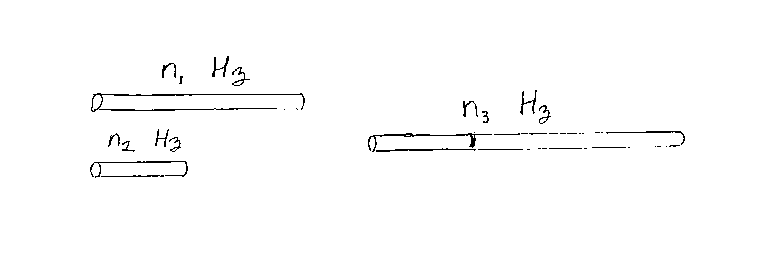Problem C19: You have two pipes that are open at each end (i.e. open-open pipes). One pipe has a fundamental frequency of n1 Hz. The other pipe has a fundamental frequency of n2 Hz. You now place the two pipes end-to-end to make one long pipe, whose length equals the sum of the lengths of the two pipes. What is the fundamental frequency of this long pipe? If the fundamental frequency equals n3 Hz, what is n3? Note that n1, n2 and n3 have the same units of frequency.n1 = n2 = Input n3:

If you are currently in my class, you can record your grade by entering your name and student ID number (without the leading zeros) below and clicking on "record grade".
 First Name = Last Name = ID = Problem: